# AN-653: Improving Temperature, Stability, and Linearity of High Dynamic Range RMS RF Power Detectors

### Abstract

Root-mean-square (rms) power detection is required to measure and control transmitted power in a multicarrier wireless infrastructure. Traditional power detectors using diode detection or log amps do not accurately measure power when the peak-to-average ratio of the transmitted signal is not fixed. Temperature stability of measurement circuitry is critical as is the linearity of the detector’s transfer function. This application note presents techniques to improve the temperature stability of an rms power detector and the linearity of its transfer function to less than ±0.3 dB over a dynamic range of more than 50 dB.

### Introduction

Modern wireless transmitters generally require strict control of transmitted radio frequency (RF) power. In wireless cellular networks, strict power control allows the size of cells to be precisely set to enhance coverage. Precise power control also avoids the need for excessive thermal dimensioning of the RF power amplifier (PA), which is required when there is uncertainty about the actual transmitted power. For example, if a 50 W (47 dBm) power amplifier has a transmit power uncertainty of just 1 dB, the PA must be dimensioned so that it can safely (i.e., without overheating) transmit 63 W (48 dBm).

Power measurement and control is also used in receivers, usually at intermediate frequencies (IFs). Here the objective is to measure and control the gain of the received signal so that IF amplifiers and analog-to-digital converters (ADCs) are not overdriven. While precision in the measurement of the received signal (commonly referred to as the received signal strength indicator or RSSI) is useful for maximizing the signal-to-noise ratio, it is less important than on the transmit side; the goal is to merely keep the received signal under a certain limit.

RMS RF power detectors can measure RF power independently of signal peak-to-average ratio or crest factor. This capability is critical when the peak-to-average ratio of a measured signal is changing. This is common in wireless cellular networks due to the ever changing number of calls being carried by a cellular base station. The changing peak-to-average ratio results both from the transmission of multiple carriers at varying power levels and from the variations in code-domain power in a single code division multiple access (CDMA) carrier.Figure 1. Modern wireless transmitters use RF power measurement and control to tightly regulate the transmitted power. In receivers, power measurement is used to prevent overdrive of IF and baseband components while maximizing signal-to-noise ratio.

### High Dynamic Range RMS-TO-DC Converter

The AD8362 is an rms-to-dc converter that can measure rms voltages over a range of 60 dB or more, spanning from very low frequencies up to approximately 2.7 GHz. Figure 2 shows the transfer function of the AD8362 at 2.2 GHz in terms of volts out versus input signal strength in dBm relative to 50 Ω.

Figure 2 also shows the deviation of this transfer function from a best fit line. This line has a slope and intercept that are calculated using a linear regression of the measured data. Once the slope and intercept of this line are calculated, an error plot, scaled in dB, can be plotted. In Figure 2, this line is scaled on the right.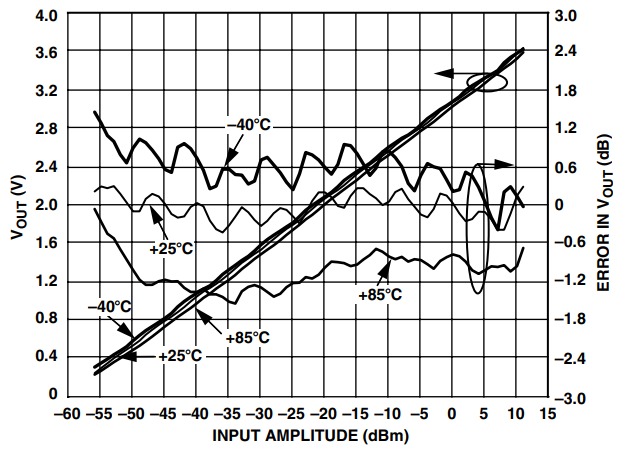Figure 2. The transfer function of a logarithmic rms-to-dc converter shows a linear-in-dB relationship between the output voltage (scaled in volts) and the dB-scaled input signal. The plot also shows transfer function ripple and temperature drift (scaled on the right axis).

This plot shows a repetitious ripple with peak-to-peak amplitude as large as 0.75 dB. This ripple results in an equally large measurement uncertainty. The plot also shows that the transfer function changes over temperature. The temperature drift of the transfer is, in this case, dominated by the change in the intercept (i.e., the slope stays relatively constant).

### Operation Of The AD8362 Logarithmic RMS-TO-DC Converter

Figure 3 shows a block diagram of the AD8362. The main components of the AD8362 are a linear-in-dB variable gain amplifier (VGA) that comprises a voltage controlled attenuator, a fixed gain amplifier, a low dynamic range rms-to-dc converter, and an error amplifier.Figure 3. AD8362 logarithmic rms-to-dc converter. The input signal to the rms-to-dc converter is applied to the VGA input. The output of the VGA is applied to the low range rms-to-dc converter. The output of this detector is compared to a setpoint voltage, which generates an error signal that feeds back to the gain control input of the VGA.

The input signal is applied to the VGA. The output of the VGA is applied to the low range rms-to-dc converter. The output of this detector is proportional to the rms voltage of the VGA output signal.

A fixed reference voltage, also referred to as the target voltage, is applied to an identical low dynamic range rms-to-dc converter. The outputs of the two detectors are applied to an error amplifier/integrator that produces an error signal. The output of the error amplifier is applied to the gain control input of the VGA. The gain control transfer function of the VGA is negative, that is, an increasing voltage decreases the gain.

When a small input signal is applied to the circuit, the voltage from the signal path detector will be small, producing a decreasing error signal that drives the VGA. This error signal will continue to decrease, increasing the VGA gain, until the output of the signal chain detector is equal to the output of the reference detector.

Likewise, a large input signal will produce an increasing error signal that decreases the gain of the VGA until the voltage from the signal path detector is equal to the reference detector. In all cases, when the system reaches equilibrium, the input voltage to the rms-to-dc converter settles to the same value. As a result, the low range rms-to-dc converter needs very little operating range for the circuit to work.

The transfer function of the VGA is linear-in-dB, that is, the gain in dB is proportional to the control voltage, scaled in volts. In this case, the slope of the VGA’s gain control is approximately –50 mV/dB. The result is a logarithmic transfer function for the overall circuit (i.e., the relationship between the input to the VGA and the output of the error amplifier), that is, the output voltage is proportional to the log or the rms input voltage. Note that the temperature stability of this gain control function is critical to the overall temperature stability of the rms measurement.

### Gaussian Interpolator

Figure 2 shows a periodic ripple in the conformance curves. The source of this ripple is the Gaussian interpolator. The Gaussian interpolator determines the nodes from which the signal is taken from the variable attenuator. This is then applied to the fixed gain amplifier that comprises the output stage of the AD8362’s VGA.

A simplified schematic of the attenuator and Gaussian interpolator circuits is shown in Figure 4. The input ladder attenuator is composed of a number of sections, each of which attenuates the input signal by 6.33 dB. The signal is tapped off these sections via variable transconductance stages. The Gaussian interpolator determines which transconductance stages are active, based upon the control signal that is applied to the control port of the variable attenuator, thereby determining the amount of attenuation applied to the input signal.Figure 4. AD8362 VGA attenuator with Gaussian interpolator. While the presence of the Gaussian interpolator yields a continuous relationship between output voltage and control voltage, the relationship has a periodic ripple.

Attenuation levels that fall between tap points require that adjacent transconductance stages be active simultaneously to produce a weighted average of these tap points according to the transconductance cell that is directed to conduct harder. The manner in which the conductance of adjacent stages changes to slide the tap point along the attenuator is responsible for the ripple observed in the conformance curves.

### Filtering Of The Error Signal

The squaring cell in the low range rms-to-dc converter produces a component at dc along with a component at twice the input frequency. This follows from the trigonometry identity

If this signal is a single-tone sine wave, the output of the squaring cell will be a dc component and a sine wave tone at twice the input frequency. The dominant pole of the error amplifier/integrator will filter out the double frequency component, leaving just the dc component.

If the input signal is a broadband signal, such as a CDMA or wideband CDMA (WCDMA) signal, the component that appears at dc spans the range from dc to half the original signal bandwidth. Therefore, once the double frequency has been filtered out, the output of the circuit that is fed back to the VGA still contains significant ripple, which appears as a noise-like signal superimposed on a dc level. Normal practice is to increase the filtering in the error amplifier so that the noise on the signal at the output of the error amplifier is significantly reduced. This results in a noise-free output from the overall circuit.

### Removal Of Transfer Function Ripple

Figure 5 shows an alternate configuration for the circuit that takes advantage of this baseband noise. In contrast to the circuit in Figure 3, the size of the integrator’s external filter capacitor has been significantly reduced but is still kept large enough to effect valid rms averaging. When a broadband signal is applied as an input to the circuit, the output of the error amplifier contains significant noise but is still centered on the correct rms output level. The noise level at the output of the error amplifier is set to a level of at least 300 mV p-p, 300 mV being the distance in dB between adjacent taps on the R-2R ladder of the VGA × the gain control slope of the VGA (i.e., 50 mV/dB × 6 dB). As long as this output noise level is at least 300 mV p-p, its actual value is not critical.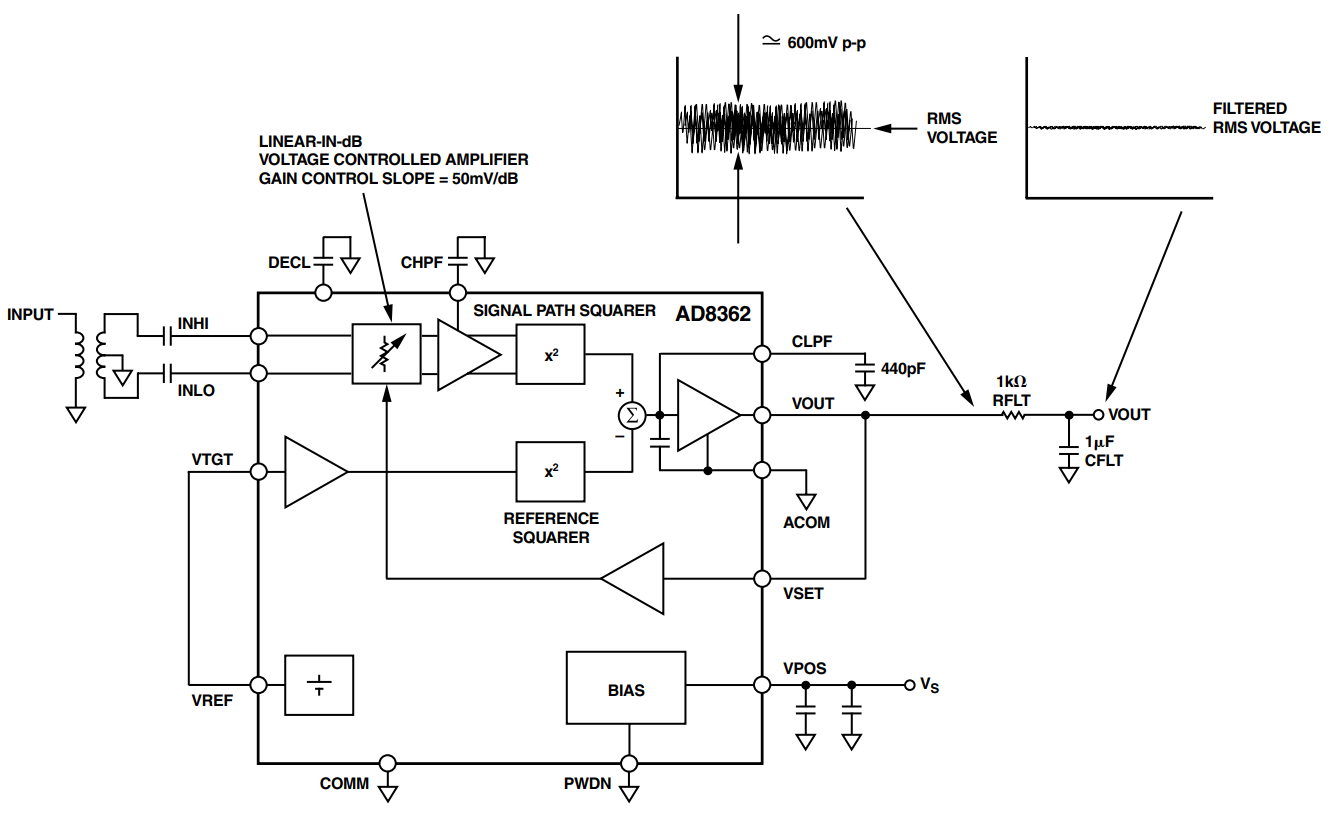Figure 5. The size of the filter capacitor, which normally works to reduce noise at the output of the squaring cell, is reduced. The noise fed back to the VGA causes the gain of the VGA to fluctuate over at least 6 dB. This tends to even out the ripple in the transfer function of the VGA, which in turn evens out the transfer function of the overall circuit. The noise at the squarer output is externally filtered before being measured.

This lightly filtered signal is fed back to the VGA control input. The noise in this signal causes the gain of the VGA to fluctuate around a central point. The gain control slope of the VGA is 50 mV/dB. As a result, the noise will cause the instantaneous gain of the VGA to change by approximately 6 dB. The wiper of the Gaussian interpolator is moved back and forth across approximately one tap of the R-2R ladder.

Because the gain control voltage is constantly moving across at least one tap of the Gaussian interpolator, the relationship between the rms signal strength of the VGA output and the VGA control voltage becomes independent of the VGA’s gain control ripple. The signal being applied to the squaring cell is now lightly AM modulated. However, this modulation does not change the peak-to-average ratio of the signal.

Because of the reduced filter capacitor, the rms voltage appearing at the output of the error amplifier now contains significant peak-to-peak noise. While it is critical to feed this signal back to the VGA gain control input with the noise intact, the rms voltage going to the external measurement node can be filtered using a simple filter to yield a largely noise-free rms voltage.

Figure 6 shows the resulting reduction in transfer function ripple of the rms-to-dc converter. The 600 mV peak-to-peak noise fed back to the VGA gain control terminal may seem needlessly large because only enough noise to exercise the gain control voltage over 6 dB (one tap on the R-2R ladder) is required. However, as the call loading in a spread spectrum CDMA signal decreases, the peak-to-average ratio of the signal also decreases. This results in less noise appearing at the detector output. As a result, the peak-to-peak noise is set so that it always spans at least one tap on the R-2R ladder. Note that the peak in the error function at around –57 dBm is due to measurement error in the high dynamic range rms power meter head that was used to measure the power being delivered to the circuit.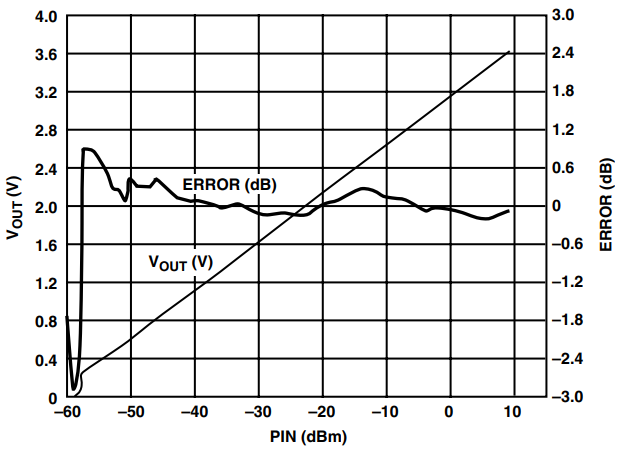Figure 6. Reduction in transfer function ripple for a high peak-to-average ratio signal (single carrier WCDMA, test model 16, 2.2 GHz). Peak at –57 dBm is due to measurement error.

Figure 7 shows the transfer function of the modified circuit when an unmodulated sine wave is applied. No reduction in transfer function ripple results. As already noted, when a sine wave is applied to the squaring cell, the output products are the double frequency and a dc voltage level. Because the sine wave is narrow band, no noise-like voltages appear close to dc. Once the double frequency has been removed, no ac component is available to exercise the VGA’s gain control input over any range.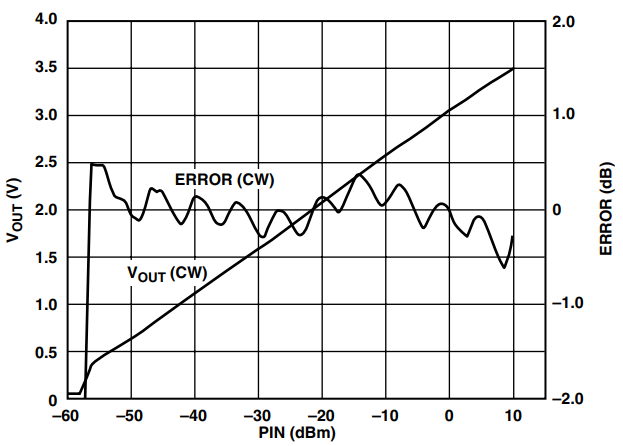Figure 7. Applying an unmodulated (2.2 GHz) sine wave to the circuit results in no reduction in the transfer function ripple because no baseband dither is produced at the output of the low range rms detector.

### Dither Injection At VTGT

Figure 8 shows an alternative circuit that can be used in these cases. The dithering signal required to exercise the VGA is coupled onto the reference voltage (also called the target voltage). This produces a disturbance at the output of the error amplifier that is fed back to the VGA gain control input. The signal that is coupled onto the VREF signal can be either noise or a coherent signal such as a sine wave.Figure 8. The dithering signal can be applied to the VTGT pin. This technique is useful if the peak-to-average ratio of the input signal is low (e.g., a sine wave). The dithering signal can be either a sine wave or white noise.

Figure 9 shows the transfer function of this circuit when a sine wave is applied as an input signal. The VTGT voltage, which is nominally 1 V dc, now has a 500 mV p-p, 10 kHz sine wave superimposed on it. Transfer function ripple reduction achieved is similar to that of the WCDMA signal. The frequency of the dithering signal is not very critical. It should be set high enough so that the output ripple can be easily filtered while achieving the desired pulse response time.Figure 9. By applying a dithering signal to the VTGT input (500 mV p-p at 10 kHz, dc level = 1 V), similar ripple reduction can be achieved with input signals with low peak-to-average ratios. In this case, the input signal is a 2.2 GHz sine wave.

### Temperature Compensation

In addition to the measurement uncertainty caused by transfer function ripple, the temperature drift of the device causes further (and greater) measurement uncertainty (Figure 2). Looking at a large population of devices (Figure 10), however, reveals that the temperature drift has a consistent trend. The output voltage increases with decreasing temperature. The amount of drift will, however, vary from part to part. Also, the magnitude of the drift varies with frequency. The Appendix shows plots of temperature drift for the population at other frequencies.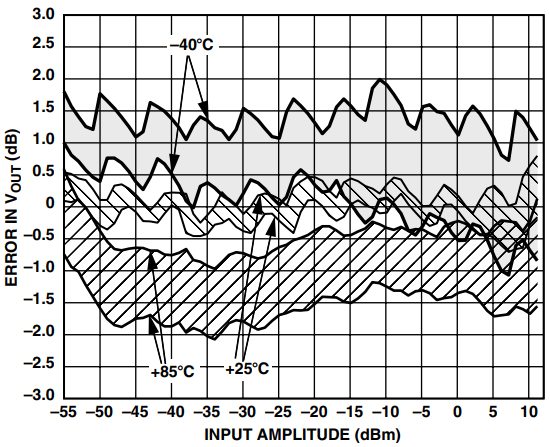Figure 10. The statistical distribution of the part-to-part temperature drift at 2.2 GHz (mean ±(3 sigma) shows output voltage consistently going high at cold and low at hot. The temperature drift is dominated by movement of the intercept.

Using the simple technique shown in Figure 11, the drift over temperature of this device can be reduced further. As already noted, the drift of the output voltage of the AD8362 is mostly due to intercept drift. The whole transfer function tends to drop with increasing temperature, while the slope remains quite stable. This makes the temperature drift fairly independent of input level. Compensating the temperature drift in this way, based upon the drift at a particular input level (e.g., 5 dBm), will hold up well over the complete dynamic range (Figure 12).Figure 11. The low temperature drift of the AD8362 can be reduced further by adding a small offset voltage with a positive temperature coefficient to the log amp’s output voltage.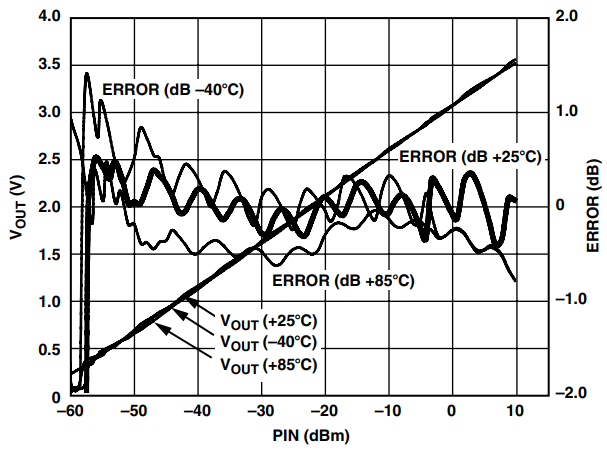Figure 12. Using a simple intercept temperature compensation scheme, the temperature drift of the AD8362 can be significantly reduced. In this case, the 2.2 GHz drift at 5 dBm is being compensated. Because the temperature drift is intercept dominated, good performance is achieved over the complete range.

The compensation scheme is simple and relies on the TMP36, a precision temperature sensor, to drive one side of the resistor divider, and the other side will be driven by the AD8362 and the output being taken at the center tap. The TMP36 has an output voltage of 750 mV at 25°C and a temperature coefficient of 10 mV/°C. As the temperature increases, the voltage from the AD8362 drops while the voltage from the TMP36 rises. R1 and R2 are chosen so that the voltage at the center of the resistor divider remains steady over temperature. In practice, R2 will be much larger than R1 so that the output voltage from the circuit will be quite close to the voltage of the AD8362’s VOUT pin.

### Choosing R1 And R2

The resistor ratio R1/R2 is determined by the temperature drift of the AD8362 at the frequency of interest. The drift at a particular input level is chosen. This yields the best precision at that level. In the example shown, R1 and R2 are chosen based upon the drift at an input level of 5 dBm. R1 and R2 are chosen according to the equation

where 10 mV/°C is the drift of the TMP36 and the AD8362 drift is specified in mV/°C. The temperature drift in dB/8C is converted to mV/°C through multiplication by the logarithmic slope. For example, the drift at 900 MHz, which is –0.008 dB/°C (at 5 dBm), is converted through multiplication by the 50 mV/dB slope to get –0.4 mV/°C. Table I shows this calculation and the resultant values for R2 and R1 for frequencies of 900 MHz, 1900 MHz, and 2200 MHz.

 Frequency (MHz) Average Drift @ 5 dBm (dB/°C) Slope (mV/dB) Average Drift @ 5 dBm (mV/dB) R1 (kΩ) R2 (kΩ) 900 –0.008 50 –0.4 mV/°C 1.02 25.5 1900 –0.0024 51 –0.1224 mV/°C 1 82.5 2200 –0.0104 50.5 –0.5252 mV/°C 1 19.1

### Composite Ripple Reduction And Temperature Compensation Circuit

The two schemes of temperature compensation and transfer function ripple reduction can be combined to yield a single, highly linear, temperature-stable rms detector.

Figure 13 shows the circuit implementation. The two compensation circuits are isolated from each other by an op amp buffer.Figure 13. The dither reduction and temperature compensation schemes can be combined to yield a single circuit with low temperature drift and excellent transfer function linearity.

Figure 14 shows the transfer function of this circuit, measured at 2.2 GHz at –40°C, +25°C, and +85°C. The measurement error is equal to approximately ±0.5 dB over a 60 dB range. As already noted, the error spike at about –57 dBm is due to the underreporting of the input signal to the AD8362 by the high dynamic range rms power meter head being used in the measurement.Figure 14. Combining the ripple reduction and temperature compensation schemes yields a circuit with measurement linearity of approximately ±0.5 dB over a range of approximately 60 dB (excessive error at low power is due to measurement error).

### Conclusion

Although the AD8362, a 60 dB logarithmic TruPwr detector, has excellent baseline performance, its measurement accuracy can be improved even further. The techniques used are simple, involve resistors, capacitors, and a temperature sensor, and can be mass produced due to the part-to-part repeatability of the temperature drift.

### Appendix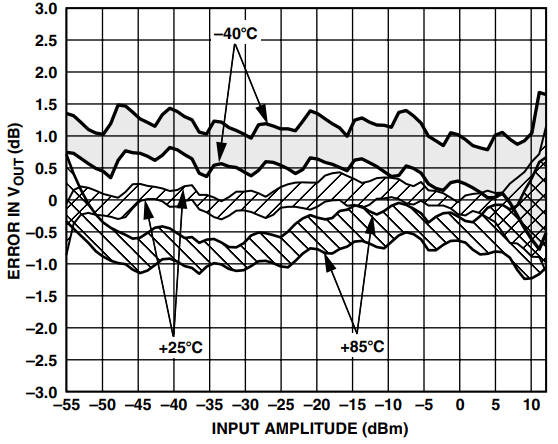Figure 15. Logarithmic Law Conformance vs. Input Amplitude, 3 Sigma to Either Side of Mean, Sine Wave, Frequency 900 MHz, Temperature –40°C, +25°C, and +85°C.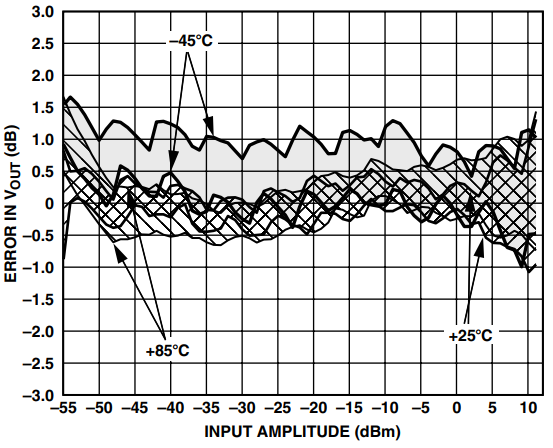Figure 16. Logarithmic Law Conformance vs. Input Amplitude, 3 Sigma to Either Side of Mean, Sine Wave, Frequency 1900 MHz, Temperature –40°C, +25°C, and +85°C.